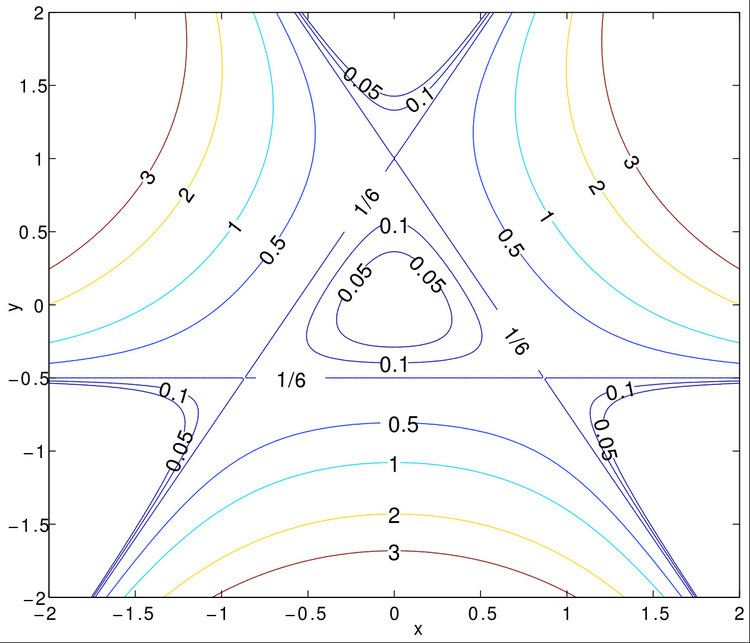# Hénon–Heiles System

Updated on
Covid-19While at Princeton in 1962, Michel Hénon and Carl Heiles worked on the non-linear motion of a star around a galactic center with the motion restricted to a plane. In 1964 they published an article titled "The applicability of the third integral of motion: Some numerical experiments". Their original idea was to find a third integral of motion in a galactic dynamics. For that purpose they took a simplified two-dimensional nonlinear axi-symmetric potential and found that the third integral existed only for a limited number of initial conditions. In the modern perspective the initial conditions that do not have the third integral of motion are called chaotic orbits.

## Introduction

The Hénon–Heiles Potential can be expressed as

V ( x , y ) = 1 2 ( x 2 + y 2 ) + λ ( x 2 y y 3 3 ) .

The Hénon–Heiles Hamiltonian can be written as

H = 1 2 ( p x 2 + p y 2 ) + 1 2 ( x 2 + y 2 ) + λ ( x 2 y y 3 3 ) .

The Hénon–Heiles system (HHS) is defined by the following four equations:

x ˙ = p x , p x ˙ = x 2 λ x y , y ˙ = p y , p y ˙ = y λ ( x 2 y 2 ) .

In the classical chaos community, the value of the parameter λ is usually taken as unity. Since HHS is specified in R 2 , we need a Hamiltonian with 2 degrees of freedom to model it. It can be solved for some cases using Painlevé analysis.

## Quantum Henon–Heiles Hamiltonian

In the quantum case the Henon–Heiles Hamiltonian can be written as a two-dimensional Schrödinger equation.

The corresponding two-dimensional Schrödinger equation is given by

i t Ψ ( x , y ) = [ 2 2 m 2 + 1 2 ( x 2 + y 2 ) + λ ( x 2 y 1 3 y 3 ) ] Ψ ( x , y ) .

## Wada property of the exit basins

Hénon–Heiles system shows rich dynamical behavior. Usually the wada property cannot be seen in the Hamiltonian system, but Hénon–Heiles exit basin shows an interesting wada property. It can be seen that when the energy is greater than the critical energy, the Hénon–Heiles system has three exit basins. In 2001 M. A. F. Sanjuán et al. had shown that in the Hénon–Heiles system the exit basins have the wada property.

## References

Similar Topics
Arena (2011 film)
Ramón Ávila
Shaunaka Rishi Das
Topics